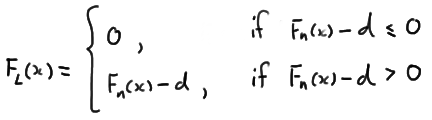# 22.3 - A Confidence Band

22.3 - A Confidence Band

Another application of the Kolmogorov-Smirnov statistic is in forming a confidence band for an unknown distribution function $$F(x)$$. To form a confidence band for $$F(x)$$, we basically need to find a confidence interval for each value of x. The following theorem gives us the recipe for doing just that.

Theorem

A $$100(1−\alpha)\%$$ confidence band for the unknown distribution function $$F(x)$$ is given by $$F_L(x)$$ and $$F_U(x)$$ where d is selected so that $$P(D_n ≥ d) = \alpha$$ and:and:### Proof

We select d so that:

$P(D_n \ge d)=\alpha$

Therefore, using the definition of $$D_n$$, and the probability rule of complementary events, we get:

$P(sup_x|F_n(x) -F(x)| \le d) =1-\alpha$

Now, if the largest of the absolute values of $$F_n (x) - F(x)$$ is less than or equal to d, then all of the absolute values of $$F_n (x) - F(x)$$ must be less than or equal to d. That is:

$P\left(|F_n(x) -F(x)| \le d \text{ for all } x\right) =1-\alpha$

Rewriting the quantity inside the parentheses without the absolute value, we get:

$P\left(-d \le F_n(x) -F(x) \le d \text{ for all } x\right) =1-\alpha$

And, subtracting $$F_n (x)$$ from each part of the resulting inequality, we get:

$P\left(- F_n(x)-d \le -F(x) \le -F_n(x)+d \text{ for all } x\right) =1-\alpha$

Now, when we divide through by −1, we have to switch the order of the inequality, getting:

$P\left(F_n(x)-d \le F(x) \le F_n(x)+d \text{ for all } x\right) =1-\alpha$

We could stop there and claim that:

$F_n(x)-d \le F(x) \le F_n(x)+d \text{ for all } x$

is a $$100(1−\alpha)\%$$ confidence band for the unknown distribution function $$F(x)$$. There's only one problem with that. It is possible that the lower limit is less than 0 and it is possible that the upper limit is greater than 1. That's not a good thing given that a distribution function must be sandwiched between 0 and 1, inclusive. We take care of that by rewriting the lower limit to prevent it from being negative:and by rewriting the upper limit to prevent it from being greater than 1:As was to be proved!

Let's try it out an example.

## Example 22-4Each person in a random sample of n = 10 employees was asked about X, the daily time wasted at work doing non-work activities, such as surfing the internet and emailing friends. The resulting data, in minutes, are as follows:

108 112 117 130 111 131 113 113 105 128

Use the data to find a 95% confidence band for the unknown cumulative distribution function $$F(x)$$.

As before, we start by ordering the x values. The formulas for the lower and upper confidence limits tell us that we need to know d and $$F_n (x)$$ for each of the 10 data points. Because the $$\alpha$$-level is 0.05 and the sample size n is 10, the table of Kolmogorv-Smirnov Acceptance Limits in the back of our text book, that is, Table VIII, tells us that $$d = 0.41$$. We already calculated $$F_n (x)$$ in the previous example.

Now, in calculating the lower limit, $$F_L(x) = F_n (x) − d = F_n (x)−0.41$$, we see that the lower limit would be negative for the first four data points:

0.1−0.41 = −0.31 and 0.2−0.41 = −0.21 and 0.3−0.41 = −0.11 and 0.4−0.41 = −0.01

Therefore, we assign the first four data points a lower limit of 0, and then just calculate the remaining lower limit values. Similarly, in calculating the upper limit, $$F_U (x) = F_n (x) + d = F_n (x) + 0.41$$, we see that the upper limit would be greater than 1 for the last six data points:

0.6+0.41 = 1.01 and 0.7+0.41 = 1.11 and 0.8+0.41 = 1.21 and 0.9+0.41 = 1.31 and 1.0+0.41 = 1.41

Therefore, we assign the last six data points an upper limit of 1, and then just calculate the remaining upper limit values. To summarize,The last two columns together give us the 95% confidence band for the unknown cumulative distribution function $$F(x)$$.

  Link ↥ Has Tooltip/Popover Toggleable Visibility